## Example Questions

← Previous 1

### Example Question #1 : How To Find The Solution To An Inequality With Addition

Solve |x – 5| ≤ 1

4 ≤ x ≤ 6

0 ≤ x ≤ 1

None of the answers are correct

x ≤ 4 or x ≥ 6

-1 ≤ x ≤ 1

4 ≤ x ≤ 6

Explanation:

Absolute values have two answers:  a positive one and a negative one.  Therefore,

-1 ≤ x – 5≤ 1 and solve by adding 5 to all sides to get 4 ≤ x ≤ 6.

### Example Question #1 : Inequalities

Solve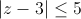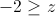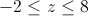All real numbers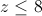No solutionsExplanation:

Absolute value is the distance from the origin and is always positive.

So we need to solve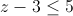and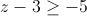which becomes a bounded solution.

Adding 3 to both sides of the inequality givesand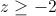or in simplified form### Example Question #3 : How To Find The Solution To An Inequality With Addition

Given the inequality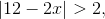which of the following is correct?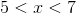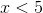or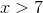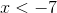or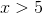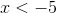or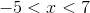orExplanation:

First separate the inequality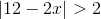into two equations.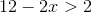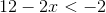Solve the first inequality.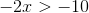Solve the second inequality.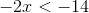Thus,or.

### Example Question #11 : Inequalities

What values of x make the following statement true?

|x – 3| < 9

–3 < x < 9

–6 < x < 12

6 < x < 12

x < 12

–12 < x < 6

–6 < x < 12

Explanation:

Solve the inequality by adding 3 to both sides to get x < 12.  Since it is absolute value, x – 3 > –9 must also be solved by adding 3 to both sides so: x > –6 so combined.

### Example Question #20 : Inequalities

If –1 < w < 1, all of the following must also be greater than –1 and less than 1 EXCEPT for which choice?

3w/2

w2

|w|

|w|0.5

w/2

3w/2

Explanation:

3w/2 will become greater than 1 as soon as w is greater than two thirds. It will likewise become less than –1 as soon as w is less than negative two thirds. All the other options always return values between –1 and 1.

### Example Question #21 : Inequalities

Solve for.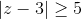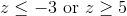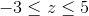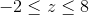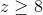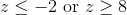Explanation:

Absolute value problems always have two sides: one positive and one negative.

First, take the problem as is and drop the absolute value signs for the positive side: z – 3 ≥ 5. When the original inequality is multiplied by –1 we get z – 3 ≤ –5.

Solve each inequality separately to get z ≤ –2 or z ≥ 8 (the inequality sign flips when multiplying or dividing by a negative number).

We can verify the solution by substituting in 0 for z to see if we get a true or false statement. Since –3 ≥ 5 is always false we know we want the two outside inequalities, rather than their intersection.

### Example Question #28 : Inequalities

If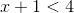and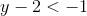, then which of the following could be the value of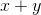?Explanation:

To solve this problem, add the two equations together: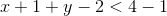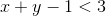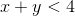The only answer choice that satisfies this equation is 0, because 0 is less than 4.

### Example Question #22 : Inequalities

What values ofmake the statement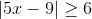true?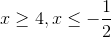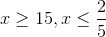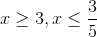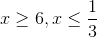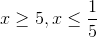Explanation:

First, solve the inequality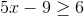: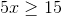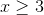Since we are dealing with absolute value,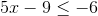must also be true; therefore: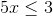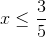### Example Question #4 : How To Find The Solution To An Inequality With Addition

Simplify the following inequality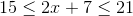.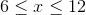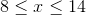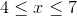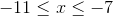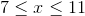Explanation:

For a combined inequality like this, you just need to be careful to perform your operations on all the parts of the inequality. Thus, begin by subtractingfrom each member: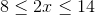Next, divide all of the members by:### Example Question #5 : How To Find The Solution To An Inequality With Addition

Simplify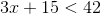.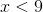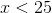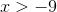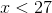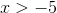Explanation:

Simplifying an inequality like this is very simple. You merely need to treat it like an equation—just don't forget to keep the inequality sign.

First, subtractfrom both sides: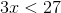Then, divide by:← Previous 1